# Motion problems - 8th grade (13y) - math problems

#### Number of problems found: 178

• Cars motion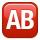From point A car start at the speed of 90 km/h. Against it departs from the point B at the same time another car at 60 km/h. For how long and where they met if the distance between A and B is 180 km?
• Bob traveled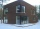Bob traveled 20 meters to Dave's house. He arrived in 3 minutes. What was his average speed for the trip.
• Motion 2At 10 o'clock started from place A car speed at 90 km/h. 20 minutes after started from the place B at speed 60 km/h another car. Distance places A and B is 120 kilometers. What time and where they meet?
• RPMAn electric motor makes 3,000 revolutions per minutes. How many degrees does it rotate in one second?
• The swimmerThe swimmer swims at a constant speed of 0.85 m/s relative to water flow. The current speed in the river is 0.40 m/s, the river width is 90 m. a) What is the resulting speed of the swimmer with respect to the tree on the riverbank when the swimmer motion
• Motion problemFrom Levíc to Košíc go car at speed 81 km/h. From Košíc to Levíc go another car at speed 69 km/h. How many minutes before the meeting will be cars 27 km away?
• Free fall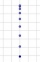For how long and at what speed does the body fall to the ground during a free fall from a height of 35 m?
• MotionFrom two different locations distant 232 km started against car and bus. The car started at 5:20 with average speed 64 km/h. Bus started at 7:30 with average speed 80 km/h. When they meet? How many kilometers went the bus?
• An airplaneAn airplane flies 1440 km in 2 1/4 hours. What is its average speed in km per hour?
• Runners circle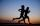Pepa circles the track in 36 seconds. Kamil in 42 seconds. They started together. How many seconds will meet again at the start?
• Free fall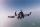Lloyd fall from height 7 m. Calculate the speed he hit the ground when falling with acceleration g = 9.81 m/s2
• Two boats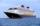The sightseeing boat sail at 25 km / h. The cruise take 6 hours. How long the another boat sails at 60 km/h?
• Hiker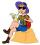Hiker went half of trip the first day, the third of trip the second day and remaines 15 km. How long trip he planned?
• The train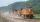The train passed the observer in the 20s and passed through a 1500m tunnel in 2 minutes. Calculate the speed (km/h) and train length.
• Tractor wheels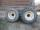Smaller tractor wheel has a circumference of 3 meters, bigger 4 meters. On what path executes smaller wheel 6 turns over more bigger?
• Runner 2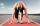Marian run 12 meters in 8 seconds. How far would Marian run for 70 seconds, if still run at the same pace?
• Taxi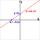A taxi ride costs \$3 plus \$2.50 per mile. Write and Graph an equation in two variables that represents the total cost of a taxi ride.
• Timber logThe ship goes from point x to y. Downstream it takes 4 hours, upstream 6 hours. How long does it take from a point x to y a log?
• Passenger car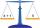Passenger car travels 1 km for 2.3 minutes, a truck during a 50 seconds long. How much is the speed of the truck less?
• Distance runner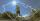Distance runner already climbed three fifths of the total route. Thus passed 90 km. How many km he have to stop? How long is his route?

Do you have an interesting mathematical word problem that you can't solve it? Submit a math problem, and we can try to solve it.

We will send a solution to your e-mail address. Solved examples are also published here. Please enter the e-mail correctly and check whether you don't have a full mailbox.

Please do not submit problems from current active competitions such as Mathematical Olympiad, correspondence seminars etc...

Motion problems - math word problems. Examples for 8th grade.